# How to Extract Text before @ Sign from an Email Address in Excel?

• Last Updated : 21 Aug, 2021

You got a dataset and you want to extract the name part of the email address. This task can be done with a text to column and formula that uses the LEFT and FIND functions.

## Using LEFT and FIND functions

### The LEFT function:

The LEFT function returns a given text from the left of our text string based on the number of characters specified.

Syntax:

`LEFT(text, [num_chars])`

Parameters:

• Text: The text we want to extract from.
• Num_chars (Optional): The number of characters you want to extract. Default num_chars is 1 and the number must be a positive number that is greater than zero.

Example:

Step 1: Format your data.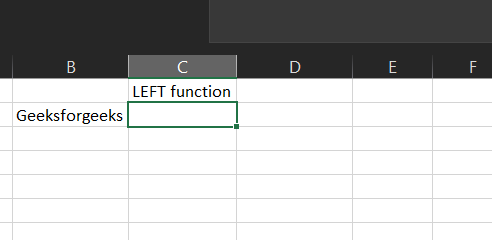Now if you want to get the first geeks from geeksforgeeks in B2. Let us follow the next step.

Step 2: We will enter =LEFT(B2,5) in the B3 cell. Here we want Excel to extract the first 5 characters from the left side of our text in B2.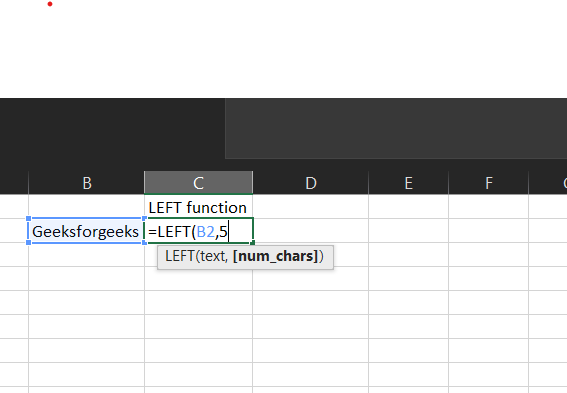This will return Geeks.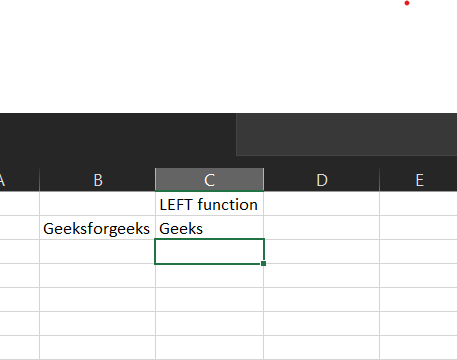Let us move to the FIND function.

### The FIND function:

The FIND function returns the position of a given text within a text.

Syntax:

`FIND(find_text, within_text, [start_num])`

Parameters:

• Find_text: The text we want to find.
• Within_text: The text containing our find_text.
• Start_num (Optional): The starting position of our find_text. Default is 1.

Example:

Step 1: Format your data.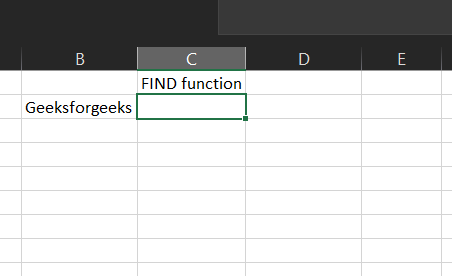Now if you want to find “for” in geeksforgeeks in B2. Let us follow the next step.

Step 2: We will enter =FIND(“for”,B2) in B3 cell. Here we want Excel to find “for” in our text in B2.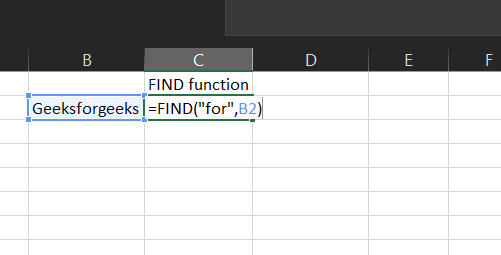This will return 6 because “for” is located at character number 6 in our text.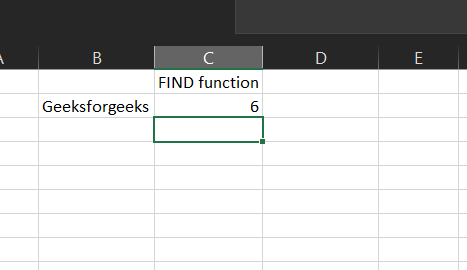Now let’s use both to extract text before the “@” in an email address.

Step 1: Format our data.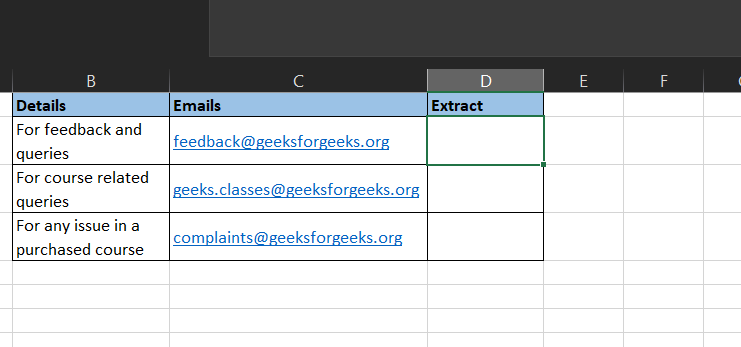Step 2: Let’s find the location of the “@” from the email. Here we use the FIND function.

We will enter =FIND(“@”,C2) in C3 cell. Here we want Excel to find “@” in our text in C2.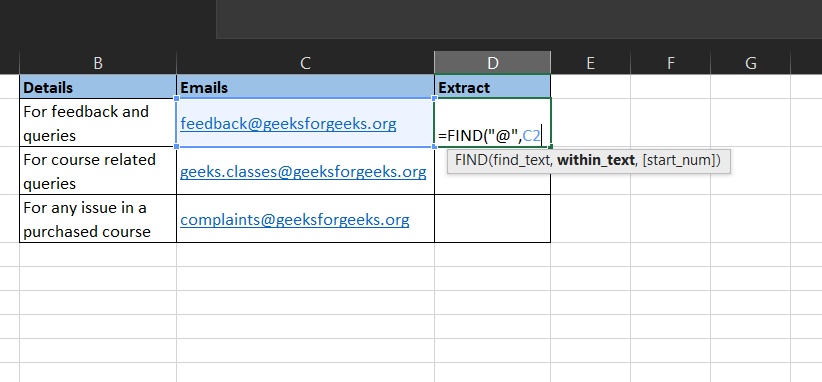Step 3: This will return 9. Next, because we want the text and not the number, we would use the LEFT function while utilizing the location function of the FIND function.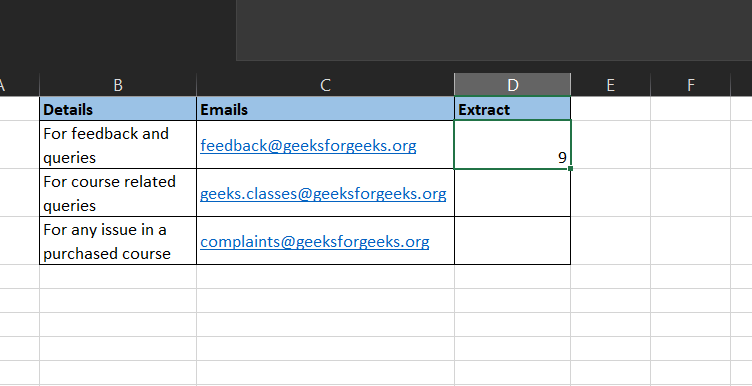Step 4:  We will enter =LEFT(C2,FIND(“@”,C2)) in the C3 cell. Here we want Excel to extract the number of characters defined by our FIND function from the left side of our text in C2.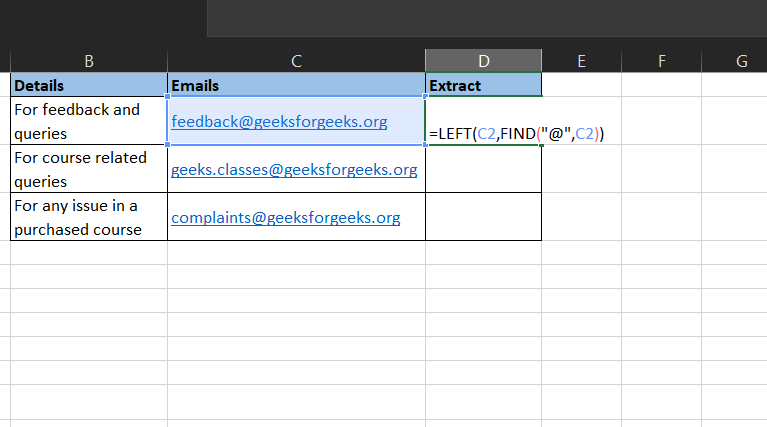Step 5: But this returns a “feedback@”.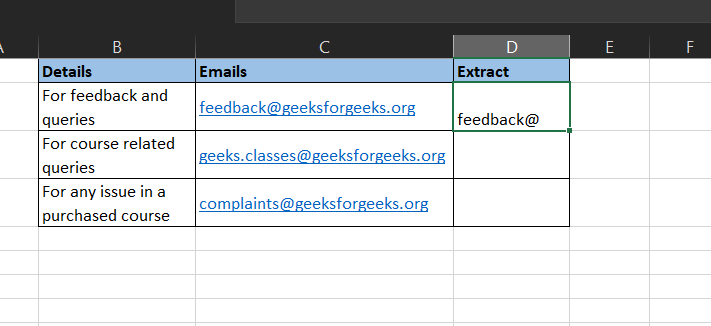Step 6: Now we need to modify the formula. Thus we add a -1 so we get the text exactly right before the “@”.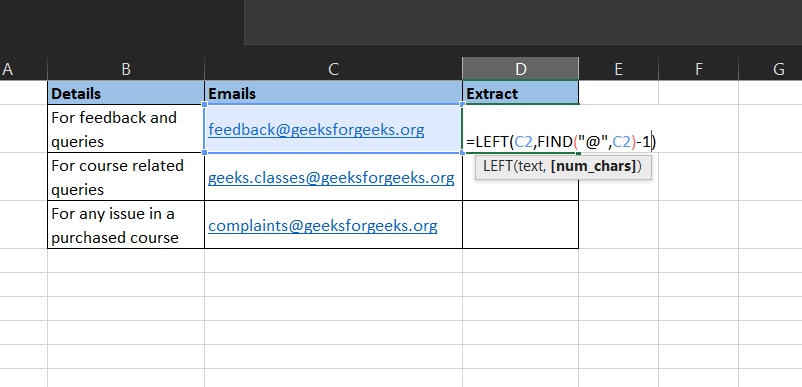Step 7: This returns what we want “feedback”. Now we can drag the formula down.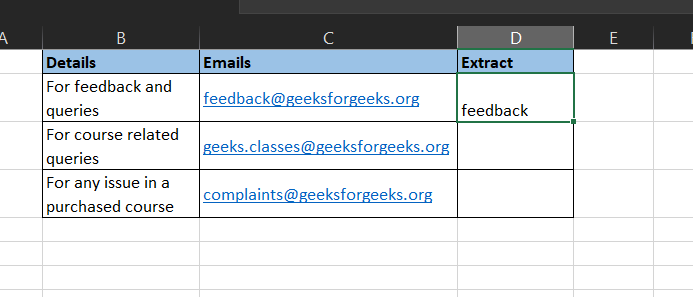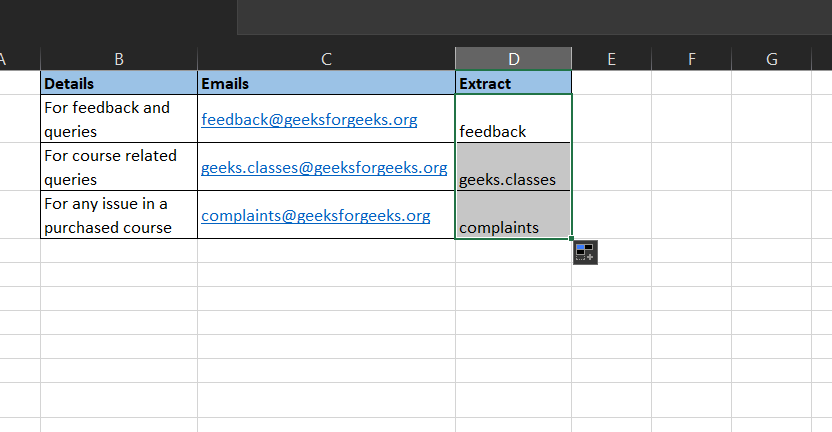## Using Text to columns

This would extract it using the delimiter aspect of the text to column features of excel:

Step 1: We format our data.Step 2: Select column C, which contains the email address.

Step 3: Move to the Data ribbon and click on Text to Columns.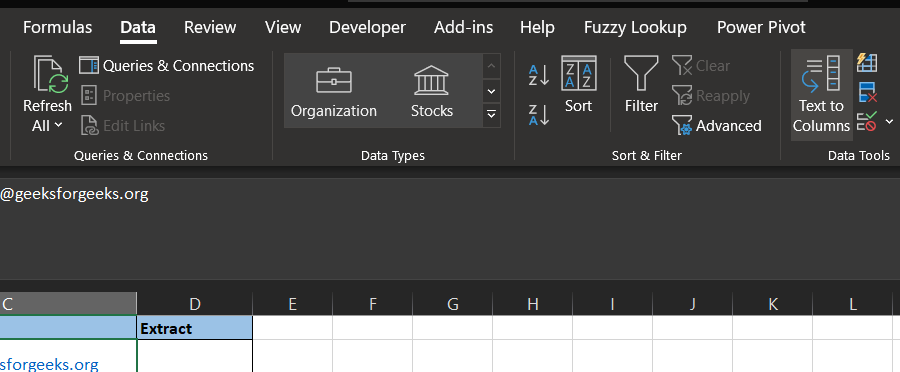Step 4: The Text to column box pops up. Though, the default is often Delimited, select Delimited if it is not. Click on Next.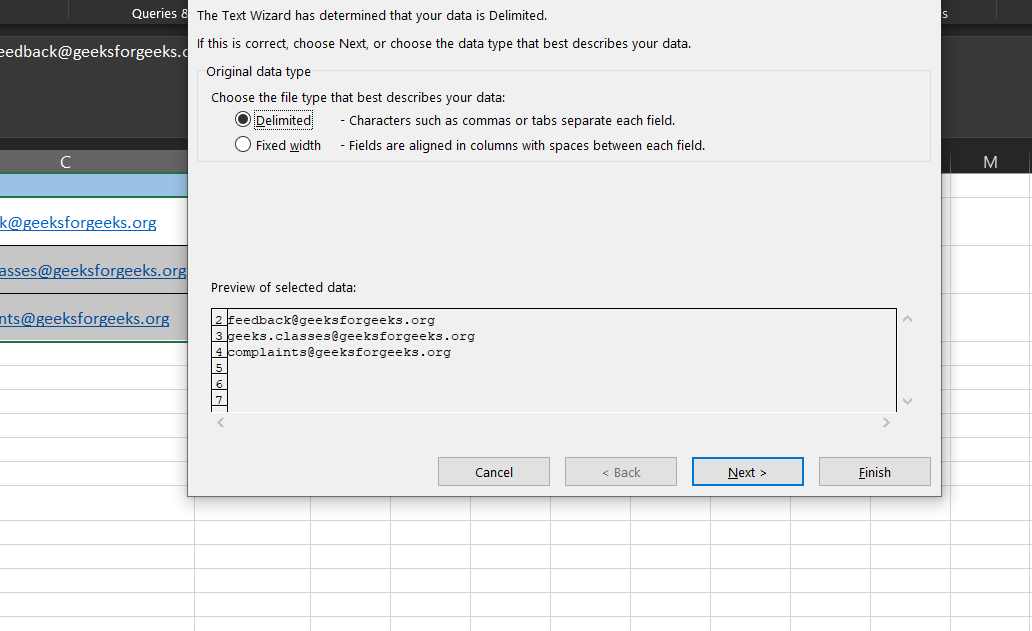Step 5: Untick the tab, tick the other check box and write down “@”. Click on Next.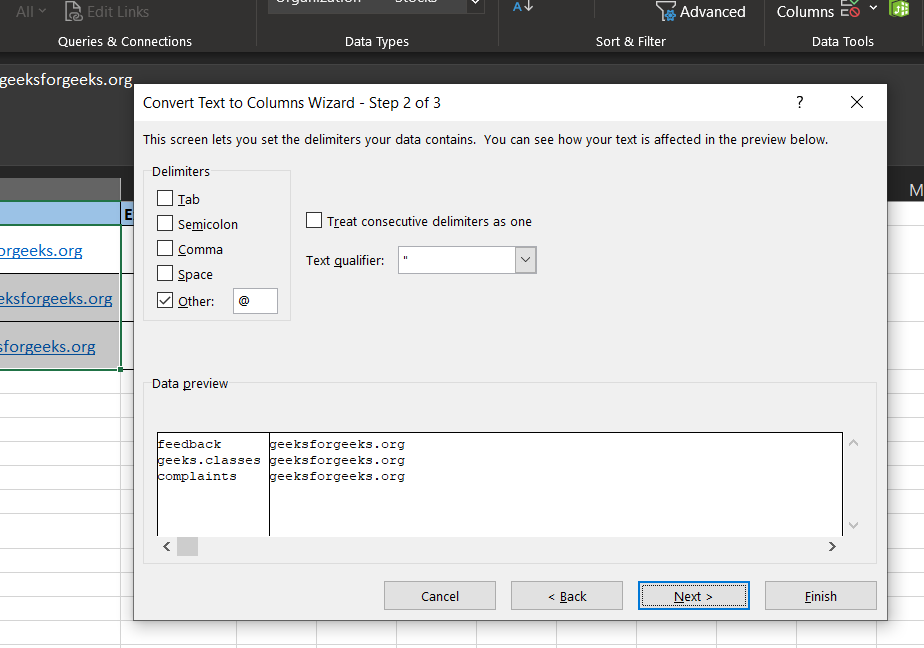Step 6: Specify your location. We would change the \$C\$2 to \$D\$2 because we want the result in cell D2. Click on Finish.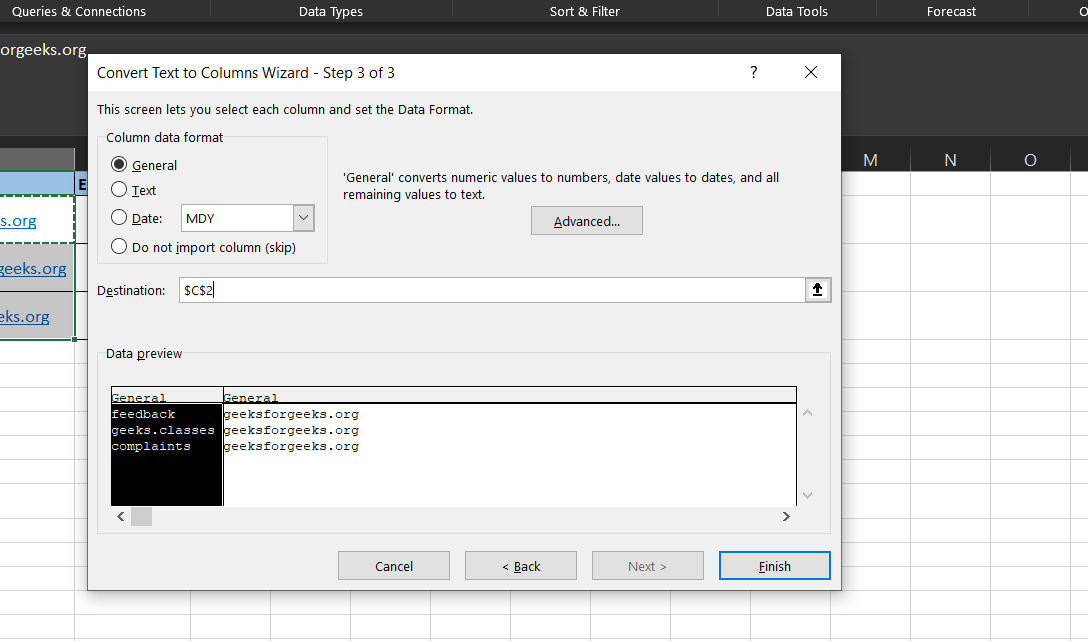Step 7: We delete the content in the E column.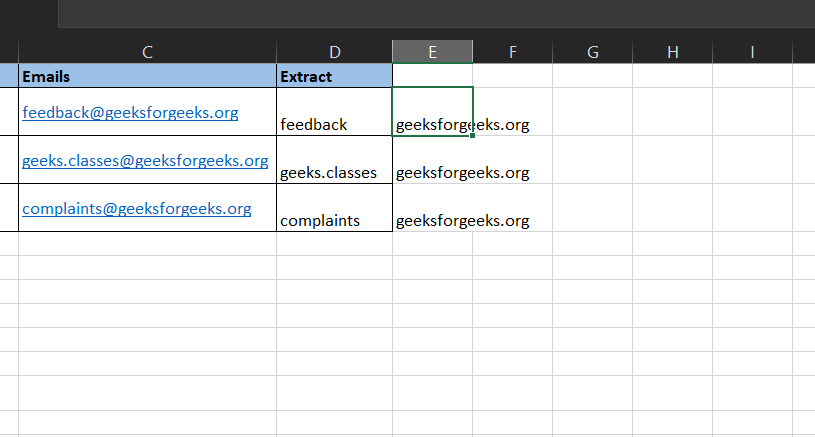Step 8: Viola! We have our text before the “@”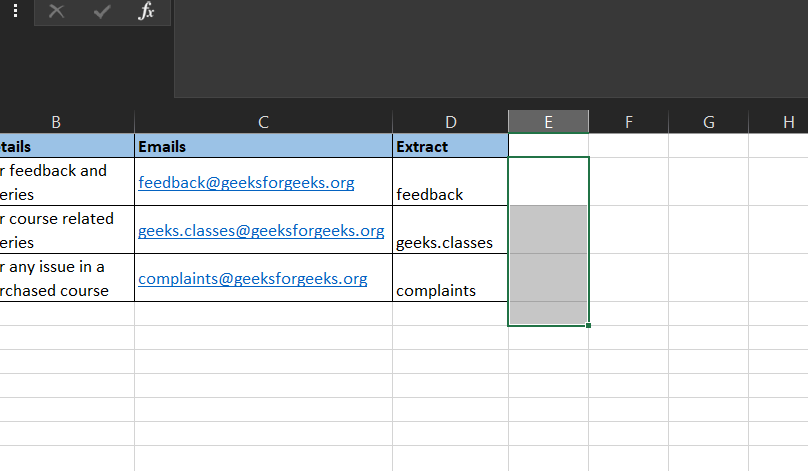My Personal Notes arrow_drop_up
Recommended Articles
Page :